Select PageIf you are an investor, finding your own stocks to buy or making your own stock picks can be difficult to do.

Lets face it, evaluating stocks to buy and sell can be tough, even with a company’s financial information available at your fingertips. There are a variety of stock market ratios and metrics out there that can give the financial health of a company, some of them are very useful.

Here are five financial ratios that can tell you most of what you need to know when you’re in search of good stocks that can be added to your portfolio.

## 1. Price-Earnings Ratio (P/E)

Price to Earnings ratio is probably the most commonly used and most well known ratio when it comes to evaluating stocks.

The PE ratio is a valuation of a company’s current stock price compared with its latest full-year earnings per share. The most frequently used earnings number in the calculation is the total earnings per share over the past four reported quarters. You could also use “forward” earnings, which is the average of Wall Street’s forecasts for the current fiscal year.

The PE ratio simply takes the stocks current share price and is divided by the latest earnings per share.

Calculated as: Share Price / Earnings per Share (EPS) = P/E Ratio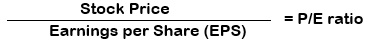The P/E ratio is a helpful measure to tell you how undervalued or overvalued a stock is. Typically, the lower the ratio, the cheaper the stock.

But, the PE ratio shouldn’t be a final determinant to tell you whether the stock is undervalued or not. Many companies that are growing at a fast rate will typically have a higher P/E ratio because higher premiums are given to faster growing companies.

For individual stocks, a good rule of thumb is you should compare a stock’s P/E with those of its competitors.

The P/E ratio range varies between sectors/indexes. For example, the small cap index will likely have a higher P/E ratio than the large cap index as the expected growth rate is higher.

If it’s lower than average, it’s a sign that you’ve spotted a possible bargain, but that all depends on growth, which leads us into the next ratio to watch, the PEG ratio.

## 2. Price/Earnings Growth (PEG) Ratio

The PEG Ratio is similar to the PE Ratio, but it takes it one step further by accounting for future earnings growth.

The PEG ratio calculation uses the basic format of the P/E ratio for a numerator and then divides by the potential growth for EPS, ideally the next five years’ worth, however some calculate it based on the growth rate for the next 12 months.

Calculated as: (P/E Ratio) / Projected Annual Growth in Earnings per Share = PEG Ratio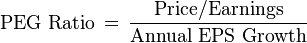For example, if a company has a P/E of 25 and is growing at 30% a year, it would have a PEG of .83 (25/30 = .83).

Typically, a PEG ratio of less than 1.0 implies that the stock is undervalued and the market is not fully valuing the prospects for future growth.

The downside of the PEG ratio is that future growth rates are sometimes hard to predict. Some companies’ growth profiles can change considerably over time.

## 3. Price/Cash Flow (P/CF)

Price/cash flow ratio is another investment valuation ratio used by investors to evaluate the attractiveness of investing in a company’s shares.

The price-to-cash flow ratio (P/CF) is a measure used to compare a company’s market value to its cash flow. The ratio is calculated in a similar manner to the price-to-earnings ratio with one exception—price per share is divided by operating cash flow as opposed to earnings per share

It is calculated by dividing the per-share stock price by the per-share operating cash flow. It can also be calculated by dividing the company’s market cap by the company’s operating cash flow in the most recent fiscal year (last four fiscal quarters).

Calculated as: Share price / cash flow per share = Price-to-Cash Flow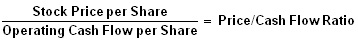The general rule of thumb is that the lower a stock’s price/cash flow ratio is, the better value that stock is.

Some investors prefer to use P/CF ratio over P/E ratio, as it is hard to manipulate cash figures as compared to “earnings (EPS)” figure which can easily be manipulated or can be affected by non cash items, for example, Depreciation, Amortization. Despite all these considerations, P/E ratio is still widely used and a recognized method of investment valuation.

## 4. Price-to-Sales (P/S)

The P/S reflects how much investors are paying for every dollar in annual sales. Essentially, the P/S number reveals the value placed on sales by the market.

The Price-to-Sales ratio is calculated by dividing the company’s market cap by total sales over the past 12 months. It can also be calculated by dividing the per-share stock price by the per-share revenue

Calculated as:
(Market Capitalization) / (Total Sales) = P/S or (Stock Price) / (Sales Per Share) = P/S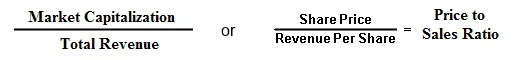Typically when calculated, the P/S used the “trailing twelve months”, which is the reported sales for the previous four quarters, although longer time periods can be examined.

The lower the P/S, the better the value since the investor is paying less for each unit of sales. However, sales do not reveal the whole picture, as the company may be unprofitable with a low P/S ratio. Because of the limitations, this ratio is usually used only for unprofitable companies, since they don’t have a P/E ratio.

## 5. Price-To-Book Value (P/BV)

Also known as price-equity ratio, the Price-To-Book Value ratio compares a stock’s market value to its book value.

The P/BV ratio basically puts a value on how much investors are paying for every dollar of assets owned by the company and gives an idea on how much money the company would be worth if the company was broken up and sold everything off.

It is calculated by dividing the current price of the stock by the latest quarter’s book value per share.

Calculated as: Share Price / Book Value = P/B ratio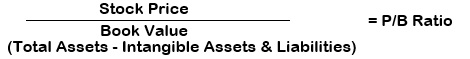Typically, a lower P/B ratio means that the stock is undervalued. However, P/B ratio is most useful for comparing companies within asset-intensive businesses but service-oriented businesses or those without substantial property, plant and equipment typically have little book value and trade at very high P/BV multiples.

For example, a mining company such as Freeport-McMoRan (NYSE:FCX) will trade at a much lower P/BV ratio compared to Facebook (NASDAQ:FB) because a mining company is a more asset-intensive business. As with most ratios, be aware that this varies by industry. The best comparisons are within industries and against a company’s historical ratios.